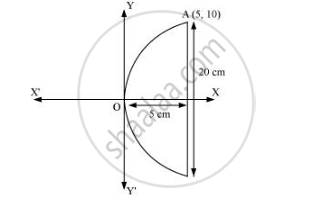CBSE (Arts) Class 11CBSE
Share
Notifications

View all notifications
Books Shortlist
Your shortlist is empty

# If a Parabolic Reflector is 20 Cm in Diameter and 5 Cm Deep, Find the Focus. - CBSE (Arts) Class 11 - Mathematics

Login
Create free account

Forgot password?
ConceptParabola Standard Equations of Parabola

#### Question

If a parabolic reflector is 20 cm in diameter and 5 cm deep, find the focus.

#### Solution

The origin of the coordinate plane is taken at the vertex of the parabolic reflector in such a way that the axis of the reflector is along the positive x-axis.

This can be diagrammatically represented asThe equation of the parabola is of the form y2 = 4ax (as it is opening to the right).

Since the parabola passes through point A (10, 5), 102 = 4a(5)

⇒ 100 = 20a

a = 100/20 = 5

Therefore, the focus of the parabola is (a, 0) = (5, 0), which is the mid-point of the diameter.

Hence, the focus of the reflector is at the mid-point of the diameter.

Is there an error in this question or solution?

#### APPEARS IN

Solution If a Parabolic Reflector is 20 Cm in Diameter and 5 Cm Deep, Find the Focus. Concept: Parabola - Standard Equations of Parabola.
S